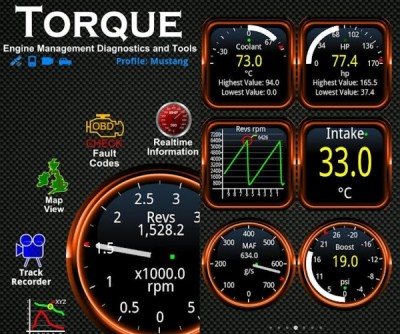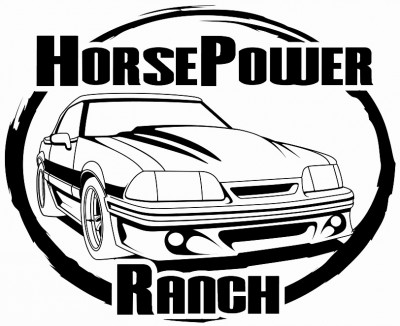# Difference Between Torque and Horsepower

Torque is one of the main characteristics of the force. To define it, you need to know the force acting on the body, the point of its applications to the body and the point of rotation of the body. Torque may be measured by knowing the power which develops in the engine.

To find the force of the torque, you can use a dynamometer. If you know the force developed by the rotating mechanism, the torque can be found by knowing its speed. It is also possible to find the torque by measuring the work done engine.

Horsepower, on the other hand, is a unit of measurement of power, firmly entrenched in the automotive industry. Horsepower is the main parameter to calculate the power of the car. Typically, this value is specified in the technical documents of the vehicle.

But sometimes it happens that the calculation of horsepower is defined in kilowatt per hour. Or, for example, the machine has passed a certain upgrade, after which its capacity increased.

### Instructions

• 1

Torque:

Find the point of application of force and the point around the body. Measure the distance between them. This will be the moment arm. Determine the direction of the force at the point of application, using a dynamometer. Using a protractor, find the acute angle between the line.

Find the torque by multiplying the value of the force and the acute angle between them (M = F • l • sin (α)). If the force is perpendicular to the point of her shoulder, then sin (α) = 1, and under these conditions develops maximum torque.

Engine performing torque is the algebraic sum of many moments of the forces acting on the various parts of the engine. Therefore, the easiest option is to find out the torque of the technical documentation that came with your device.

If such information is not available to measure the frequency of a tachometer motor shaft speed in revolutions per minute, measure the power of the engine, or learn from the documentation, expressing it in kilowatts.

Image Courtesy: androidauthority.com• 2

Horsepower:

The easiest way to find the number of horsepower in the engine of the car is to look at data sheet of the machine. If the registration card is missing, you can go to the directory corresponding to the automaker, which tells the power of all cars produced by a given manufacturer. If the power is shown in kilowatts, you can convert it by using the formula: power in kilowatts multiplied by 1.35962.

Image Courtesy: hpranch.com•